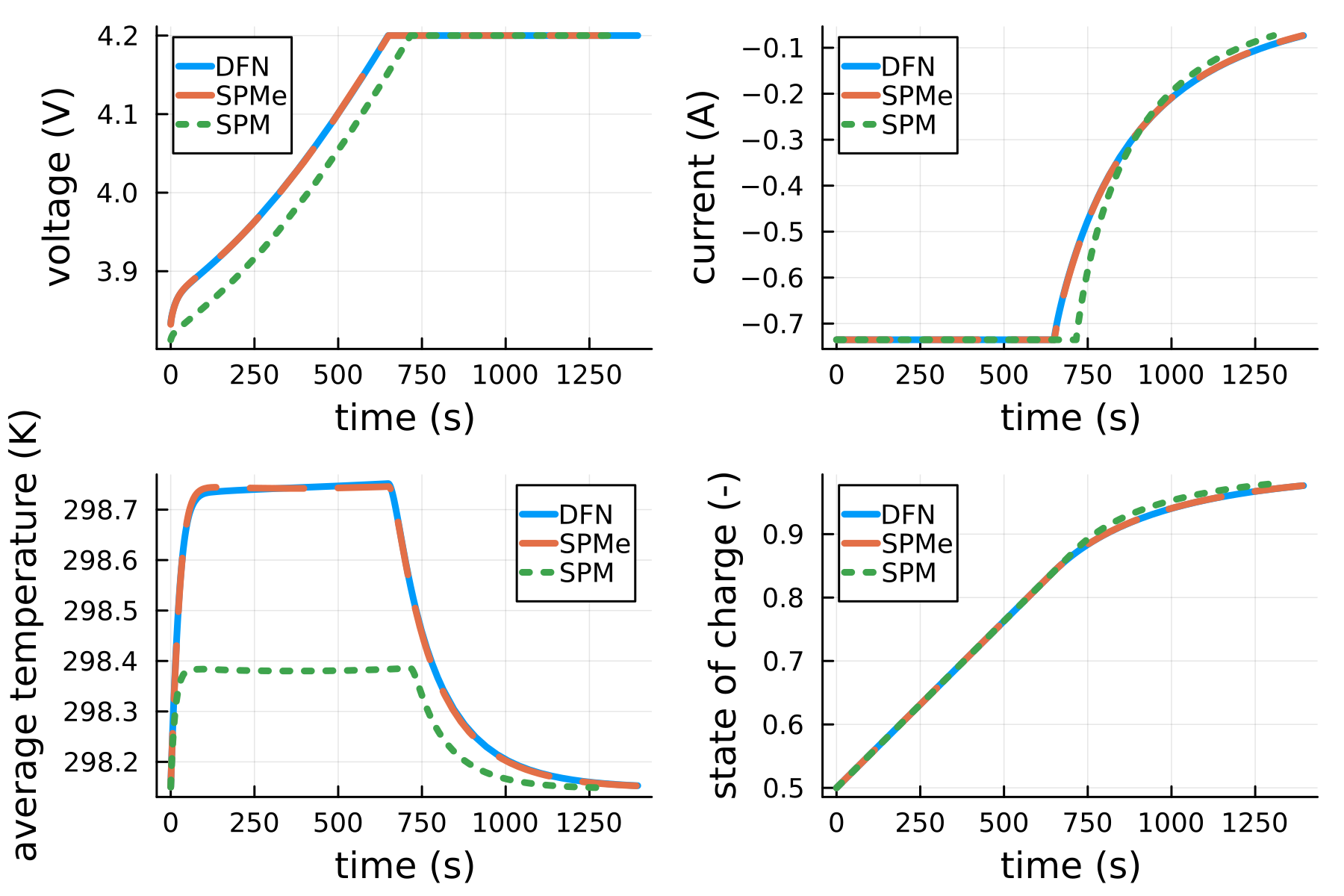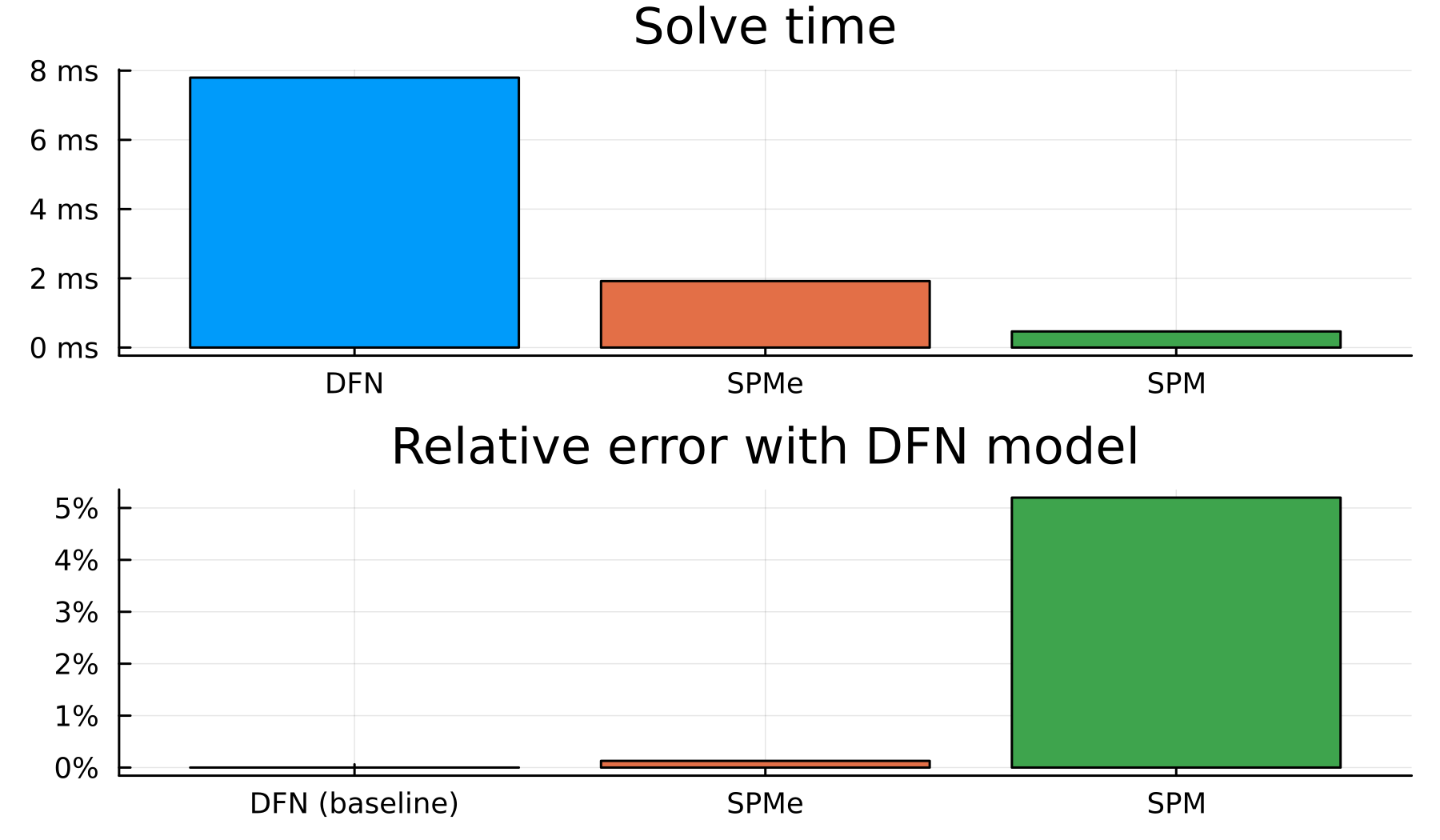### Choosing an appropriate model

JuliaSimBatteries offers three battery models, each with different levels of complexity. When selecting the best model for your application, consider the trade-offs between accuracy and computational efficiency:

• Doyle-Fuller-Newman (DFN) Model:
• Most complex and detailed representation
• Provides spatial information on both electrode and electrolyte
• Ideal for applications requiring high accuracy and detailed information
• Single-Particle Model with Electrolyte (SPMe):
• Intermediate level of complexity
• Accounts for electrolyte dynamics
• Suitable for applications that need electrolyte behavior without the full spatial detail of the DFN model
• Single-Particle Model (SPM):
• Least complex and most computationally efficient
• Simplified representation of the battery
• Best for applications where basic performance prediction is sufficient

Select the most appropriate model for your application based on your accuracy requirements and available computational resources, keeping in mind this sliding scale of complexity.

## Model comparison during fast-charge

using JuliaSimBatteries, Plots

experiment = [
charge(2C)
voltage(:hold)
]

models = [DFN, SPMe, SPM]

styles = [:solid, :dash, :dot]

outputs = ["voltage", "current", "average temperature", "state of charge"]

plts = [plot() for _ in 1:length(outputs)]

for (model, style) in zip(models, styles)
cell = model(NMC())

sol = solve(cell, experiment)

for (output, plt) in zip(outputs, plts)
plot!(plt, sol, output;
label = model, style = style, linewidth = 3)
end
end
plot(plts...)

For the NMC parameter set, the SPMe is in great agreement with the DFN model. The SPM has good agreement with the DFN model, but there are visual differences in the plots of voltage, current, average temperature, and state of charge below due to the simplifying assumptions of the model.Every unique application of battery models has different requirements for accuracy and computational efficiency. The bar plots below show the average error of the SPMe and SPM models compared to the DFN model for a range of parameter sets. Generally, the more physics in the model, the slower the simulation.## Doyle-Fuller-Newman (DFN)

The DFN model is a physics-based electrochemical model for lithium-ion batteries that accounts for:

• transport of lithium ions and electrons within the electrolyte and electrode particles
• Intercalation reaction kinetics at the electrode-electrolyte interface
• Conservation of charge in both electrodes
• 1D temperature dynamics in the cell and current collectors

This model captures the spatial variations in concentration, potential, and current density, providing a more accurate representation of the battery behavior under various operating conditions. The DFN model is preferred over the SPM when a higher level of accuracy is required, particularly for predicting battery performance, degradation, and thermal behavior.

To simulate using the DFN, run

cell = DFN(parameter_dict)

using a defined parameter_dict.

## Single-Particle Model with Electrolyte (SPMe)

The SPMe is an intermediate electrochemical model for lithium-ion batteries that incorporates the key features of both the SPM and the DFN model. Like the SPM, the SPMe represents the electrodes as single spherical particles, assuming uniform lithium concentration and potential within each particle. The SPMe accounts for:

• Lithium diffusion within the active materials
• Intercalation reaction kinetics at the electrode-electrolyte interface
• Transport of lithium ions within the electrolyte, similar to the DFN model
• 1D temperature dynamics in the current collectors

Although the SPMe does not capture the full spatial variations in concentration, potential, and current density like the DFN model, it provides a balance between computational efficiency and accuracy. The SPMe is well-suited for applications where the inclusion of electrolyte dynamics is important but computational resources are limited, such as in battery management systems and preliminary design optimization.

To simulate using the SPMe, run

cell = SPMe(parameter_dict)

using a defined parameter_dict.

## Single-Particle Model (SPM)

The SPM is a simplified electrochemical model for lithium-ion batteries that captures the essential features of battery behavior while reducing computational complexity. The SPM represents the electrodes as single spherical particles, and it assumes uniform lithium concentration and potential within each particle. The model focuses on:

• Lithium diffusion within the active materials
• Intercalation reaction kinetics at the electrode-electrolyte interface
• 1D temperature dynamics in the current collectors

Although the SPM does not account for spatial variations in concentration, potential, and current density as the DFN model does, it is computationally more efficient and is well-suited for applications where a reasonable trade-off between accuracy and computational cost is desired, such as real-time control and optimization tasks.

To simulate using the SPM, run

cell = SPM(parameter_dict)

using a defined parameter_dict.